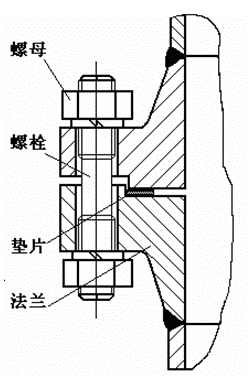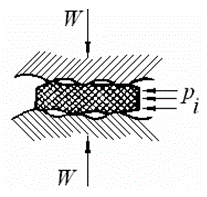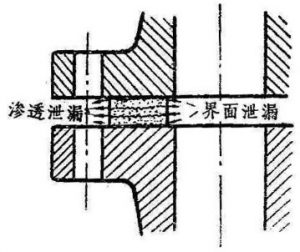### 单位换算表

#### 法兰联接结构及其密封原理##### 泄漏形式与密封原理##### 预紧密封比压和垫片系数1.预紧密封比压y

2.垫片系数m

Sg/p=m
m值被称为垫片系数。垫片（环）的材料与结构不同，预紧密封比压y值和垫片系数m的大小也不同。

#### 法兰垫片密封及密封设计

##### 一、压紧面的选择
1. 平面形压紧面常用于压力不高的场合。2. 凹凸形压紧面的垫片便于对中，但宽度较大，需较大的螺栓预紧力。3. 榫槽形压紧面用于压力更高、密封要求严的场合，但拆卸比较困难。4. 梯形槽压紧面用于较高压力场合。##### 三、螺栓的设计
1. 螺栓载荷计算

1) 在预紧工况时
$$W_a = 3.14D_G by$$

Y — 垫片的预紧比压，MPa
$$D_G$$ — 密封面平均直径，mm

2）在操作工况时
$$W_p=\frac{π}{4}D_G^2 p + 2bπD_G mp$$ 式中 $$W_p$$ — 预紧工况下的螺栓载荷，N
m — 垫片系数，
p — 设计压力，MPa
2. 螺栓尺寸与数目

$$A_a ≥ \frac{W_a}{[σ]_b} mm^2$$在操作工况：
$$A_p ≥ \frac{W_p}{[σ]_b^t} mm^2$$式中〖[σ] 〗_b^t为设计温度下螺栓材料的许用应力,MPa 螺栓所需的总截面积取上述工况下的大值
2)螺栓尺寸与数目

1) 螺栓设计载荷

$$W = \frac{(A_m+A_m)}{2} [σ]_b N$$操作工况：
$$W = W_p$$
2) 螺栓材料